Previous: Reynolds number Up: What is Turbulence? Next: Friction Coefficient

This is an automatically generated documentation by LaTeX2HTML utility. In case of any issue, please, contact us at info@cfdsupport.com.

## Turbulent Boundary Layer

The turbulent flow near solid boundaries is described by empirically-determined relationships called the Law of the wall. Measurements show, that for turbulent flows, the velocity near the wall varies logarithmically with the distance from the surface. From dimensional analysis23.2 we obtain the law of the wall: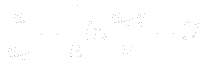(23.8)

where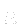is Kármán’s constant and the quantity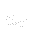is known as the friction velocity: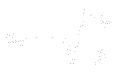(23.9)

Correlation of measurements indicate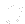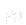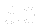and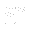.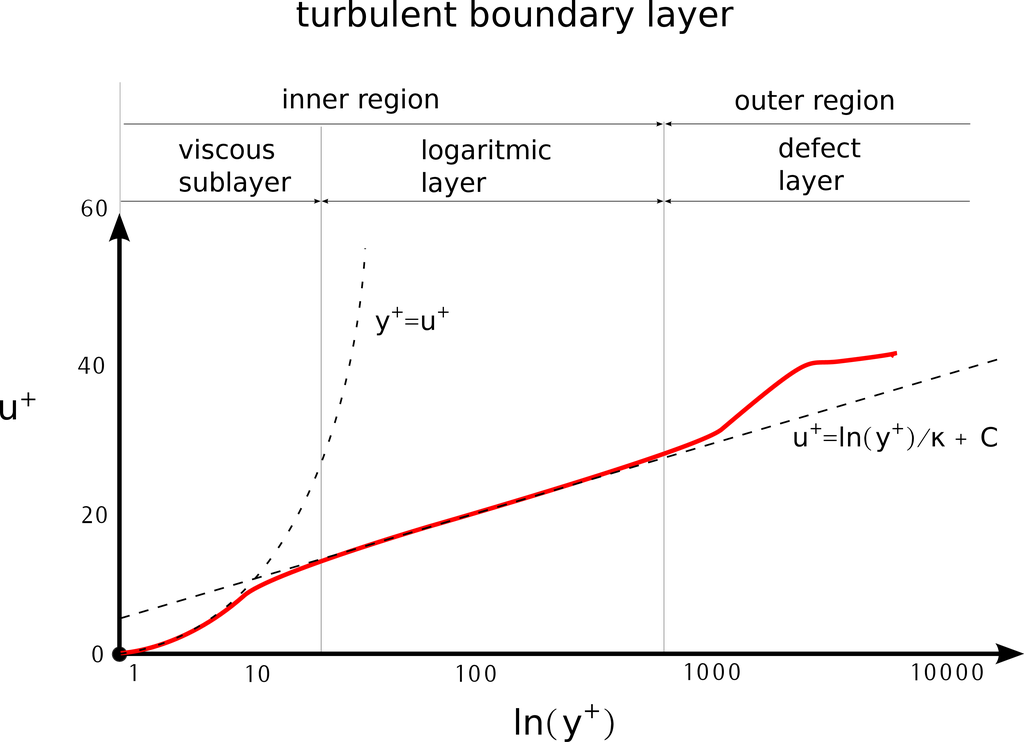Figure: A sketch of developed turbulent boundary layer in dimensionless coordinates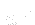and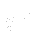.

Figure () shows typical velocity profile for a turbulent boundary layer. The graph displays the dimensionless velocity, and dimensionless wall distance, defined as: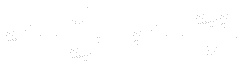(23.10)

Finally, the Law of the wall can be described: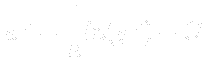(23.11)

The fully developed boundary layer can be viewed as divided into four regions:
Viscous sublayer is a region closest to the wall. In this region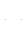The fluid flow is always laminar here. The Viscous sublayer region is typically where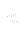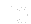.

Buffer layer is a region, where neither law holds. The Buffer layer region is typically where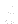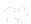.

Logarithmic (Log-law) layer is a region, where the Law of the wall holds. The Log-law layer region is typically where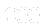.

Outer (Defect) layer is a region, where the free-stream is taking over. The Outer layer region is typically where.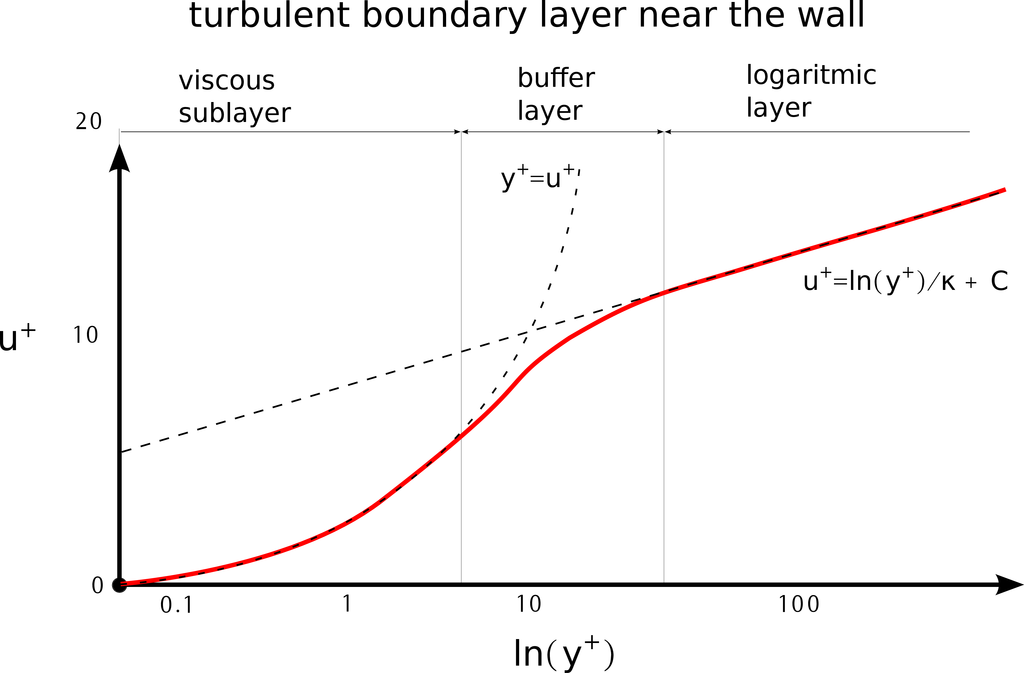Figure: A sketch of near wall detail of developed turbulent boundary layer in dimensionless coordinatesand.